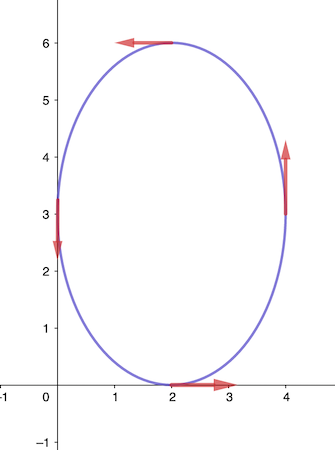# A clown is juggling at a circus. The path of a ball is given by the parametric equations x = 2...

## Question:

A clown is juggling at a circus. The path of a ball is given by the parametric equations x = 2 cos t + 2 and y = 3 sin 5 + 3. In what direction is the ball moving?

a) up and to the right

b) counterclockwise

c) down and to the right

d) clockwise

## Parametric Equations

A parametric equation given by a vector function of a single parameter, {eq}\displaystyle x=x(t), y=y(t) {/eq} has a direction,

described by the trace on the curve when the value of {eq}\displaystyle t {/eq} is increasing.

If it is a circular motion, we describe the direction as counterclockwise or clockwise.

The direction of the curve is obtained by the direction of the tangent vector to the curve or

by tracing the direction from one point to another one, obtained for a larger value of {eq}\displaystyle t. {/eq}

To determine the direction of the ball thrown by a clown, that follows the motion of the parametric curve {eq}\displaystyle x= 2\cos t+2, y=3\sin t+3, {/eq}

we will determine the tangent vector to the curve, by writing the motion of the ball as a vector function, first {eq}\displaystyle \mathbf{r}'(t)=\left\langle 2\cos t+2, 3\sin t+3 \right\rangle. {/eq}

The tangent vector is {eq}\displaystyle \mathbf{r}'(t)=\left\langle \frac{d}{dt}(2\cos t+2), \frac{d}{dt}(3\sin t+3) \right\rangle =\left\langle -2\sin t, 3\cos t \right\rangle. {/eq}

So, starting at {eq}\displaystyle t=0: \mathbf{r}'(0)=\left\langle 0, 3\right\rangle, \displaystyle \text{ going to }t=\frac{\pi}{2}: \mathbf{r}'\left(\frac{\pi}{2}\right)=\left\langle -2, 0\right\rangle, \\ {/eq}

so the motion is counterclockwise.

Starting from the lowest point on the curve, corresponding to {eq}\displaystyle t=\frac{3\pi}{2}: (x,y)=(2,0), {/eq}

the next point is obtained for {eq}\displaystyle t=2\pi: (x,y)=(4, 3) {/eq} so the motion is up and to the right.

Therefore, the motion is {eq}\displaystyle \boxed{\text{ (a) up and to the right and (b)counterclockwise }}, {/eq}

as shown in the picture by the red arrows, the velocity vector direction.